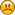###Show Posts

This section allows you to view all posts made by this member. Note that you can only see posts made in areas you currently have access to.

### Messages - dfix

Pages: 
1
##### Support / Re: calcAngle returns "NaN"
« on: May 02, 2017, 12:05:14 pm »
Yes, that makes sense,

I have an array of "jellyfish" that randomly move around the world, however, once they are a certain distance away, I need them to come back into the centre of the camera view.  I'm struggling to see how I can derive the correct rotation that points to the centre of the camera view.(the camera is static)

Code: [Select]
`private void animate()    {        if (aniFrame > 0)        {            ind += INC_FRACTION * aniFrame;            if (ind > 1) {                ind -= 1;                aniFrame = 0;            }            for (int i = 0; i < jellyFishAmount; i++)            {                jellyFishClones[i].animate(ind);                //apply the new animation frame                movedDistance[i] += MOVE_SPEED;                 //increase the directional change                if (roam[i]&&aniFrame%2==0)                     //only change direction every second call and if the jellyfish hasn't left the "safe area"                {                    jellyFishClones[i].rotateX(random.nextFloat()*0.5f);                    jellyFishClones[i].rotateY(random.nextFloat()*0.5f);                }                if (centerVec.distance(jellyFishClones[i].getTranslation()) > 200f)                {                    if (roam[i])                    {                        jellyFishClones[i].rotateX(centerVec.calcAngle(jellyFishClones[i].getXAxis()));                        jellyFishClones[i].rotateY(centerVec.calcAngle(jellyFishClones[i].getYAxis()));                        jellyFishClones[i].rotateZ(centerVec.calcAngle(jellyFishClones[i].getZAxis()));                        //jellyFishClones[i].clearTranslation();                        movedDistance[i] = 0;                          }                    roam[i] = false;                    if (centerVec.distance(jellyFishClones[i].getTranslation()) < 10f)                    {                        roam[i] = true;                         //set the jellyfish back to randomly roaming                    }                }                directionalVec[i] = jellyFishClones[i].getZAxis();                         directionalVec[i].scalarMul(movedDistance[i]);                             jellyFishClones[i].translate(directionalVec[i]);                       }       }}`

2
##### Support / Re: calcAngle returns "NaN"
« on: May 02, 2017, 04:24:16 am »
Changing the first vector's Z value from 0 to a negative number returns a correct value;

Code: [Select]
`                v=new SimpleVector(0f,0f,-10f);                ov=new SimpleVector(0f,0f,10f);`Now I get:

I/jPCT-AE: angle:3.1415927

which I was expecting from 0 also

Is it impossible to calculate an angle with one vector having an absolute value of 0?

3
##### Support / calcAngle returns "NaN"
« on: May 02, 2017, 04:13:53 am »
Hi, Can someone help me?

I am trying to calculate the angle between two vectors, but the only results I get are "NaN". I am sure it is something I am doing wrong...

Code: [Select]
`      v=new SimpleVector(0,0,0);ov=new SimpleVector(1,1,10);float angle = v.calcAngle(ov);Logger.log("angle:"+String.valueOf(angle));`
The Android Monitor just outputs:

I/jPCT-AE: angle:NaNPages: